Find Minimum Spanning Tree(for connected undirected graph)
Tree : connected graph without cycle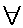u,v ,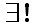a path from u to v.

Spanning Tree : edges connect all vertice of G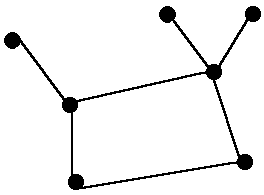LemmaV1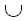V2V3V4 = V
e = (V,W) minimum edge,vVi ,w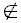Vi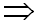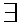MST include e
proof : (T - {e'})e
is spanning tree of Lower cost.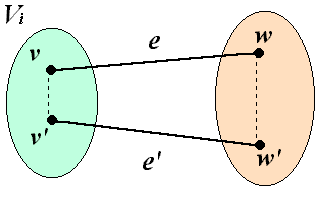Kruskal's algorithmvV , {v} a component;                                          W(e1)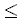W(e2).......W(eN)
repeat                                                                             for i = 1 to N doif add ei Not form cycle
Until  1  component remainsPrim's algorithm
V1 = visited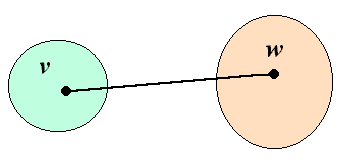V2 = unvisited

e = (v , w)
= minimal edge  visited vertex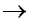fringe vertex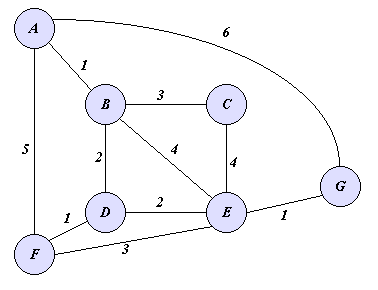Priority - First - Search   implementation : Priority = Shortest length to tr